# Excercise 5.2 Data Handling- NCERT Solutions Class 8

Go back to  'Data Handling'

## Chapter 5 Ex.5.2 Question 1

A survey was made to find the type of music that a certain group of young people liked in a city. Adjoining pie chart shows the findings of this survey.

From this pie chart, answer the following:

(i) If $$20$$ people liked classical music, how many young people were surveyed?

(ii) Which type of music is liked by the maximum number of people?

(iii) If a cassette company were to make $$1000$$ CD’s, how many of each type would they make?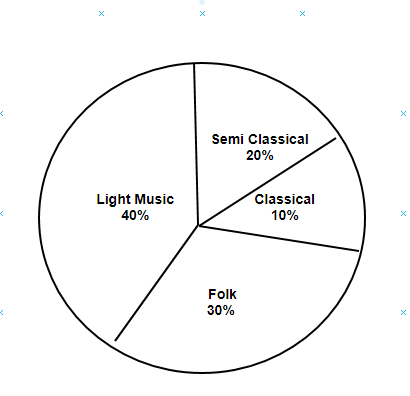### Solution

What is known?

Pie chart showing the distribution of music.

What is unknown?

(i) The number of young people were surveyed if $$20$$ people liked classical music

(ii) The type of music is liked by the maximum number of people

(iii) Each type of cassettes would they make, if a cassette company were to make $$1000$$ CD’s

Reasoning:

A circle graph or pie chart shows the relationship between a whole and its parts.

Steps:

(i) $$10\%$$ represents $$20$$ people.

Therefore, $$10\%$$ represents

\begin{align}= \frac{{100 \times 20}}{{10}} = 200\end{align} People

Hence, $$200$$ young people were surveyed.

(ii)  From the pie chart,it can be easily observed that the light music is represented by the maximum part of the pie chart $$($$i.e $$40 \%)$$Hence,light music is liked by the maximum number of people.

(iii)Number of CD’s of classical music

\begin{align}= \frac{{10 \times 1000}}{{100}} = 100\end{align}

Number of CD’s of semi-classical music

\begin{align}= \frac{{20 \times 1000}}{{100}} = 200\end{align}

Number of CD’s of light music

\begin{align}= \frac{{40 \times 1000}}{{100}} = 400\end{align}

Number of CD’s of folk music

\begin{align}= \frac{{30 \times 1000}}{{100}} = 300\end{align}

## Chapter 5 Ex.5.2 Question 2

A group of $$360$$ people were asked to vote for their favourite season from the three seasons rainy, winter and summer.

(i) Which season got the most votes?

(ii) Find the central angle of each sector.

(iii)Draw a pie chart to show this information.

 Season No. of votes Summer$$90$$ Rainy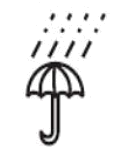$$120$$ Winter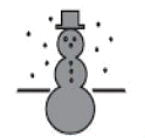$$150$$

### Solution

What is known?

Voting distribution of $$360$$ people for their favorite season.

What is unknown?

(i) The season got the most votes

(ii) The central angle of each sector

(iii) Pie chart that depicts this information

Reasoning:

A circle graph or pie chart shows the relationship between a whole and its parts.

Steps:

(i) By observing the given data,winter season got the most votes.

(ii) Central angle of summer

\begin{align} =\frac{90^{\circ} \times 360^{\circ}}{360^{\circ}}=90^{\circ} \end{align}

Central angle of rainy season

\begin{align} =\frac{120^{\circ} \times 360^{\circ}}{360^{\circ}}=120^{\circ} \end{align}

Central angle of winter season

\begin{align} =\frac{150^{\circ} \times 360^{\circ}}{360^{\circ}}=150^{\circ} \end{align}

(iii)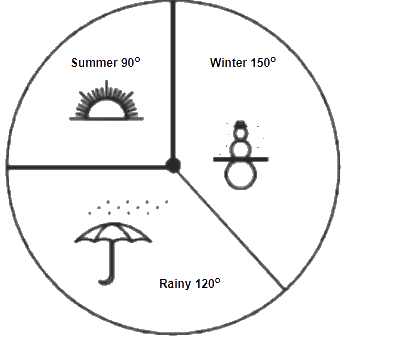## Chapter 5 Ex.5.2 Question 3

Draw a pie chart showing the following information. The table shows the colours preferred by a group of people.

 Colours No. of People Blue $$18$$ Green $$9$$ Red 6 Yellow $$3$$ Total $$36$$

### Solution

What is known?

Distribution of the colours preferred by a group of people

What is unknown?

Pie chart of the given information.

Reasoning:

A circle graph or pie chart shows the relationship between a whole and its parts.

Steps:

Here, Total angle $$= 360^\circ$$ and Total number of people $$= 36$$

The central angle for each colours can be calculated as below:

 Colours No. of People In Fraction Central Angles Blue $$18$$ \begin{align}\frac{{18}}{{36}} = \frac{1}{2}\end{align} \begin{align}\frac{1}{2} \times {360^{\rm{o}}} = {180^{\rm{o}}}\end{align} Green $$9$$ \begin{align}\frac{9}{{36}} = \frac{1}{4}\end{align} \begin{align}\frac{1}{4} \times {360^{\rm{o}}} = {90^{\rm{o}}}\end{align} Red $$6$$ \begin{align}\frac{6}{{36}} = \frac{1}{6}\end{align} \begin{align}\frac{1}{6} \times {360^{\rm{o}}} = {60^{\rm{o}}}\end{align} Yellow $$3$$ \begin{align}\frac{3}{{36}} = \frac{1}{{12}}\end{align} \begin{align}\frac{1}{{12}} \times {360^{\rm{o}}} = {30^{\rm{o}}}\end{align}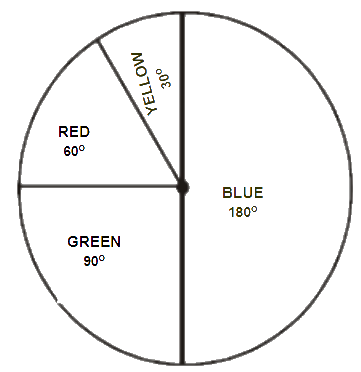## Chapter 5 Ex.5.2 Question 4

The adjoining pie chart gives the marks scored in an examination by a student in Hindi, English, Mathematics, Social Science and Science. If the total marks obtained by the students were $$540,$$ answer the following questions: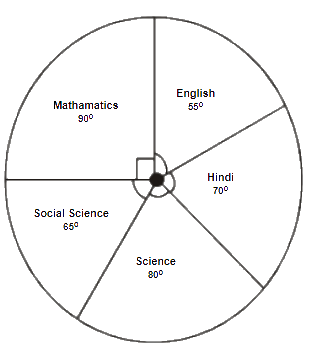(i) In which subject did the student score $$105$$ marks?
(Hint: for $$540$$ marks, the central angle$$= 360^\circ$$ a So, for $$105$$ marks, what is the central angle?)

(ii) How many more marks were obtained by the student in Mathematics than in Hindi?

(iii) Examine whether the sum of the marks obtained in Social Science and Mathematics is more than that in Science and Hindi.
(Hint: Just study the central angles)

### Solution

What is known?

(i) Pie chart describing the marks scored in an examination by a student in Hindi, English, Mathematics, Social Science and Science.

(ii) Total marks obtained by the students are $$540$$

What is unknown?

(i)  The subject in which the student scored $$105$$ marks

(ii)  Number of more marks scored by the student in Mathematics than in Hindi

(iii) Whether the sum of the marks obtained in Social Science and Mathematics is more than that in Science and Hindi.

Reasoning:

A circle graph or pie chart shows the relationship between a whole and its parts. Sum of the total angles in a pie chart is equal to $$360.$$

Steps:

 Subject Central Angle Marks obtained Mathematics $$90^\circ$$ \begin{align}\frac{{{{90}^{\circ}}}}{{{{360}^{\rm{o}}}}} \times 540 = 135\end{align} Social Science $$65^\circ$$ \begin{align}\frac{{{{65}^\circ}}}{{{{360}^{\circ}}}} \times 540 = 97.5\end{align} Science $${80^{\circ}}$$ \begin{align}\frac{{{{80}^{\circ}}}}{{{{360}^{\circ}}}} \times 540 = 120\end{align} Hindi $$70^\circ$$ \begin{align}\frac{{{{70}^{\circ}}}}{{{{360}^{\circ}}}} \times 540 = 105\end{align} English $$55^\circ$$ \begin{align}\frac{{{{55}^{\circ}}}}{{{{360}^{\circ}}}} \times 540 = 82.5\end{align}

(i) The student scored $$105$$ marks in Hindi.

(ii) Marks obtained in Mathematics $$= 135$$

Marks obtained in Hindi $$= 105$$

Difference $$= 135 – 105 = 30$$

Thus, $$30$$ more marks were obtained by the student in Mathematics than in Hindi.

(iii) The sum of marks in Social Science and Mathematics $$= 97.5 + 135 = 232.5$$

The sum of marks in Science and Hindi $$= 120 + 105 = 225$$

Yes, the sum of the marks in Social Science and Mathematics is more than that in Science and Hindi.

## Chapter 5 Ex.5.2 Question 5

The number of students in a hostel, speaking different languages is given below. Display the data in a pie chart.

 Language Hindi English Marathi Tamil Bengali Total No. of students $$40$$ $$12$$ $$9$$ $$7$$ $$4$$ $$72$$

### Solution

What is known?

Distribution of the students with respect to the language they speak.

What is unknown?

Pie chart of the given information.

Reasoning:

A circle graph or pie chart shows the relationship between a whole and its parts.Sum of the total angles in a pie chart is equal to $$360^{\circ}.$$

Steps:

The central angle for each language is calculated as below

 Language No. of students In fraction Central Angle Hindi $$40$$ \begin{align}\frac{{40}}{{72}} = \frac{5}{9}\end{align} \begin{align}\frac{5}{9} \times 360^\circ = 200^\circ \end{align} English $$12$$ \begin{align}\frac{{12}}{{72}} = \frac{1}{6}\end{align} \begin{align}\frac{1}{6} \times {360^{\circ}} = 60^\circ\end{align} Marathi $$9$$ \begin{align}\frac{9}{{72}} = \frac{1}{8}\end{align} \begin{align}\frac{1}{8} \times 360^\circ = 45^\circ\end{align} Tamil $$7$$ \begin{align}\frac{7}{{72}} = \frac{7}{{72}}\end{align} \begin{align}\frac{7}{{72}} \times {360^\circ} = {35^\circ}\end{align} Bengali $$4$$ \begin{align}\frac{4}{{72}} = \frac{1}{{18}}\end{align} \begin{align}\frac{1}{{18}} \times {360^\circ} = {20^\circ}\end{align} Total $$72$$

Pie chart for above data is drawn as below: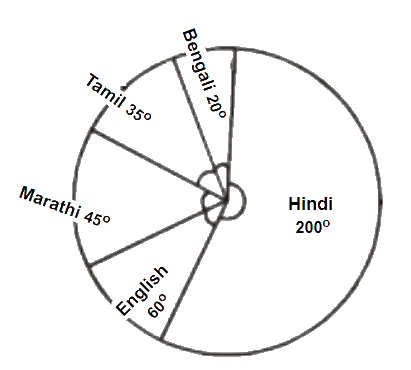Related Sections
Related Sections
Instant doubt clearing with Cuemath Advanced Math Program